Breaking News
Home / Civil Engineering / Load Calculation On Column, Beam, Wall And Slab

# Load Calculation On Column, Beam, Wall And Slab

## What Is Column ?

A compression member, i.e., column, is an important element of every reinforced concrete structure. These are used to transfer a load of superstructure to the foundation safely.

Mainly columns, struts, and pedestals are used as compression members in buildings, bridges, supporting systems of tanks, factories, and many more such structures.

A column is defined as a vertical compression member who is mainly subjected to the effective length and axial loads of which exceeds three times its least lateral dimension.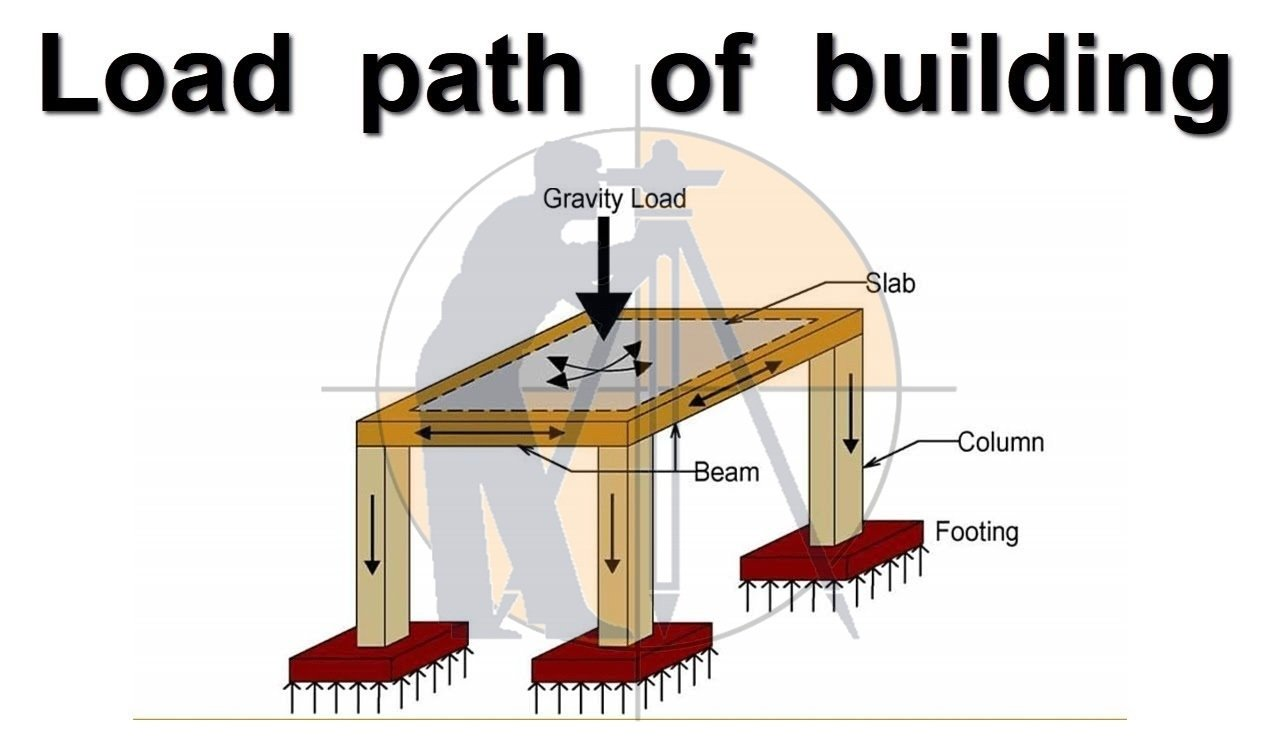The compression member whose effective length is less than three times its least lateral dimension is called Pedestal.

The compression member who is inclined or horizontal and is subjected to axial loads is called Strut. Struts are used in trusses.

3. ### Calculation Of Staircase Concrete And Bar Bending Schedule (BBS) | Staircase Reinforcement Details

The function of columns is to transfer the load of the structure vertically downwards to transfer it to a foundation. Apart from the wall performs the following functions also:

• It encloses building areas into different compartments and provides privacy.
• It provides safety from burglary and insects.
• It keeps the building warm in cools in summer and winter.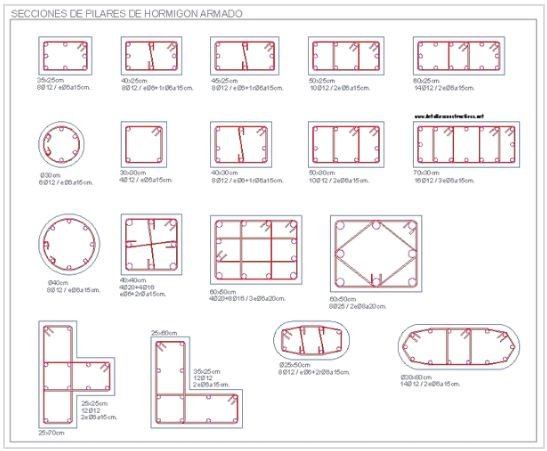## What Is Beam ?

The beam is a structural element that stands against the bending. Mainly beam carries vertical gravitational forces, but also pull the horizontal loads on it.

The beam is called a wall plate or sill plate that carries the transmits and load it to the girders, columns, or walls. It is attached with.

In the early centuries, timbers were the most preferred material to be used as a beam for this structural support purpose, now to bear the force along with carrying vertical gravitational force, now they are made up of aluminum, steel, or other such materials.

In actual means, beams are these structural materials, which bear the sheer force of the load and the bending moment.

To carry on the more tension and load, pre-stressed concrete beams are widely used nowadays in the foundation of bridges and other such humongous structures.

Several famous beams used nowadays are supported Beam, Fixed Beam, Cantilever Beam, Continuous Beam, Overhanging Beam.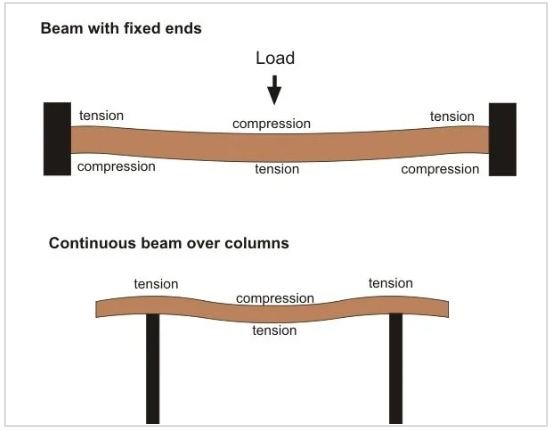## What is Wall ?

Wall is a structural element that divides the space (room) into two spaces (rooms) and also provides safety and shelter. Generally, the wall is differentiated as two types of outer-wall and inner-wall.

Outer-walls give an enclosure to the house for shelter, and inner-walls help to partition the enclosure into the required number of rooms. Inner walls are also called as Partition walls.

Walls are built to partition the living area into different parts. They impart privacy and protection against temperature, rain, and theft.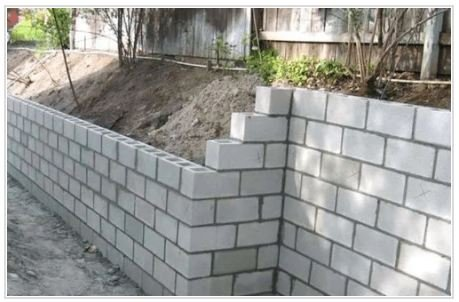## What Is  Slab ?

A slab is constructed to provide flat surfaces, typically horizontal, in building roofs, floors, bridges, and other types of structures. The slab could be supported by walls, by reinforced concrete beams normally cast monolithically with the slab, by structural steel beams, either by columns or from the ground.

A slab is a plate element having a depth (D), very small as compared to its length and width. A slab is used as floor or roof in buildings, carry distribution load uniformly.

Slab May Be

• Simply Supported.
• Continuous.
• Cantilever.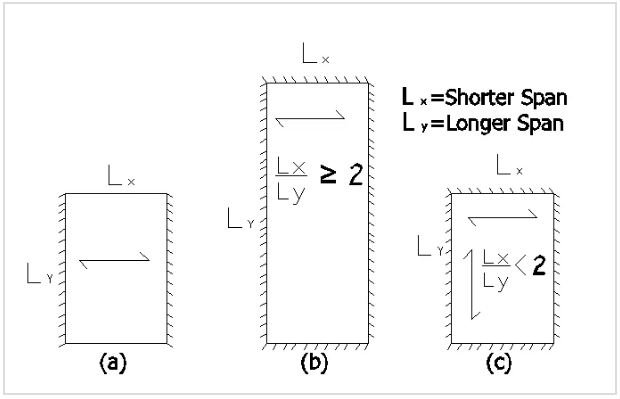## Different Load Calculation on Column, Beam, Wall And Slab

1. Column = Self  Weight x Number of floors
2. Beams = Self Weight per running meter
3. Wall Load Per Running Meter

Besides this above loading, the columns are also subjected to bending moments that have to be considered in the final design.

These tools are reduced laborious and consuming method of manual calculations for structural design, this is highly recommended nowadays in the field.

The most effective method for designing structure is to use advanced structural design software like STAAD Pro or ETABS.

for professional structural design practice, there are some basic assumptions we use for structural loading calculations.

### How to Load Calculation on Column :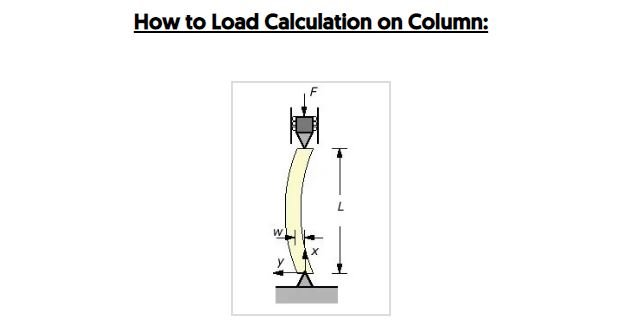### How to Create The Design Of RCC Structures With Details

we know that the Self-weight of Concrete is around 2400 kg/m3, which is equivalent to 24.54 kn/m3and the Self-weight of Steel is around 7850 kg/m3. ( Note: 1 Kilonewton Is Equal to 101.9716 Kilograms)

So, if we assume a column size of 300 mm x 600 mm with 1% steel and 2.55 (why 2.55 so, 3m column hight – beam sizemeters standard height, the self-weight of the column is around 1000 kg per floor, that id equal to 10 kN.

1. Volume of Concrete = 0.30 x 0.60 x 2.55 =0.459 m³
2. Weight of Concrete = 0.459 x 2400 = 1101.60 kg
3. Weight of Steel (1%)  in Concrete 0.459 x 1% x 7850 = 36.03 kg
4. Total Weight of Column = 1101.60 + 36.03 = 1137.63 kg = 11.12 KN

While doing calculations, we assume the self weight of columns is between 10 to 12 kN per floor.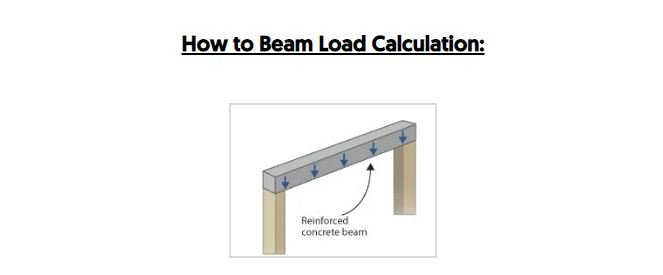### Estimating Of Steel Bar Quantity for Construction

We adopt the same method of calculations for beam also.

we assume each meter of the beam has dimensions of 300 mm x 600 mm excluding slab thickness.

Assume each (1m) meter of the beam has dimension

1. 300 mm x 600 mm excluding slab.
2. Volume of Concrete = 0.30 x 0.60 x 1 =0.18 m³
3. Weight of Concrete = 0.18 x 2400 = 432 kg
4. Weight of Steel (2%) in Concrete = 0.18 x 2% x 7850 = 28.26 kg
5. Total Weight of Column = 432 + 28.26 =  460.26 kg/m = 4.51 KN/m

So, the self-weight will be around 4.51 kN per running meter.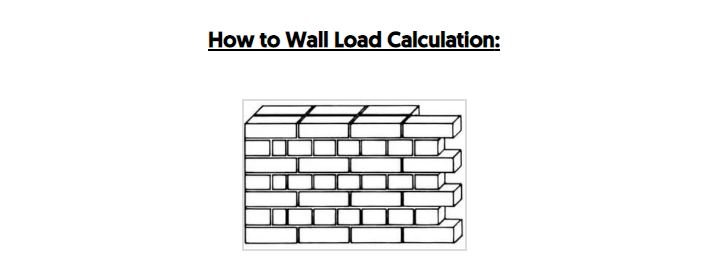### Calculation Method For Find Out The Overlapping Length Of Beam, Column And Slab

we know that the Density of bricks varies between 1800 to 2000 kg/m3.

For a 9 inch (230 mm) thick Brick wall of 2.55-meter height and a length of 1 meter,

The load / running meter to be equal to 0.230 x 1 x 2.55 x 2000 = 1173 kg/meter,

which is equivalent to 11.50 kN/meter.

This method can be adopted for load calculations of Brick per running meter for any brick type using this technique.

For aerated concrete blocks and auto-claved concrete (ACC)  blocks, like Aerocon or Siporex, the weight per cubic meter is between 550 to 650 kg per cubic meter.

The load/running meter to be equal to 0.230 x 1 x 2.55 x 650= 381.23 kg

if you are using these blocks for construction, the wall loads per running meter can be as low as 3.74 kN/meter, use of this block can significantly reduce the cost of the project.### HOW TO CALCULATE STEEL QUANTITY FOR SLAB WITH BAR BENDING SCHEDULE

Let, Assume the slab has a thickness of 150 mm.

So, the Self-weight of each square meter of the slab would be

Slab Load Calculation = 0.150 x 1 x 2400 = 360 kg which is equivalent to 3.53 kN.

Now, If we consider the Floor Finishing load to be 1 kN per meter, superimposed live load to be 2 kN per meter, and  Wind Load as per Is 875 Near about 2 kN per meter.

So, from the above data, we can estimate the slab load to be around 8 to 9 kN per square meter.

### FAQ

we know that the Self-weight of Concrete is around 2400 kg/m3, which is equivalent to 240 kN and the Self-weight of Steel is around 8000 kg/m3.

So, if we assume a column size of 230 mm x 600 mm with 1% steel and 3 meters standard height, the self-weight of the column is around 1000 kg per floor, that id equal to 10 kN.

• Volume of Concrete = 0.23 x 0.60 x 3 =0.414m³
• Weight of Concrete = 0.414 x 2400 = 993.6 kg
• Weight of Steel (1%)  in Concrete 0.414x 0.01 x 8000 = 33 kg
• Total Weight of Column = 994 + 33 = 1026 kg = 10KN

1. Density of brick wall with mortar is about ranging between 1600-2200 kg/m3. So we consider self weight of brick wall is 2200 kg/m3 in this calculation.
2. Volume of brick wall: Volume of brickwall = l × b ×h, Length = 1 meter, Width = 0.152 mm, Height of wall = 2.5 meter, Volume = 1m× 0.152 m× 2.5 m, Volume of brick wall = 0.38 m3
4. It will be converted into kilo Newton by dividing with 100 we will get 8.36 kN/m

• 300 mm x 600 mm excluding slab thickness.
• Volume of Concrete = 0.30 x 0.60 x 1 =0.18 m³
• Weight of Concrete = 0.18 x 2400 = 432 kg
• Weight of Steel (2%) in Concrete = 0.18 x 2% x 7850 = 28.26 kg
• Total Weight of Column = 432 + 28.26 =  460.26 kg/m = 4.51 KN/m

### Bar Bending Schedule Of Lintel Beam With Full Detail

column is an essential structural member of the RCC structure that helps transfer the superstructure’s load to the foundation. It is a vertical compression member subjected to direct axial load and its effective length is three times larger than its least lateral dimension.

### Dead load = volume of member x unit weight of materials.

By calculating the volume of each member and multiplying by the unit weight of the materials from which it is composed, an accurate dead load can be determined for each component.

### THANKS.